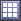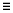# Pareto Plot Post Processing

Plot the effects of input variables on output responses in hierarchical order (highest to lowest).

## Plot the Effects of Variables on Responses in Hierarchical Order

Rank the effects of input variables on output responses in hierarchical order (highest to lowest) in the Pareto Plot post processing tab.

1. From the Post Processing step, click the Pareto Plot tab.
2. Using the Channel selector, select the response to plot.
Tip: Analyze multiple responses simultaneously by switching the Multiplot option to(multiple plots) and selecting the responses to plot using the Channel selector.
3. Analyze the pareto plot.

The effect of input variables on output responses is indicated by bars. Hashed lines with a positive slope indicates a positive effect. If an input variable increases, the output response will also increase. Hashed lines with a negative slope indicates a negative effect. Increasing the input variable lowers the output response.

A line represents the cumulative effect.

Configure the pareto plot's display settings by clicking(located in the top, right corner of the work area). For more information about these settings, refer to Pareto Plot Tab Settings.

## Pareto Plot Tab Settings

Settings to configure the plots displayed in the Pareto Plot post processing tab.

Access settings from the menu that displays when you click(located above the Channel selector).
Effect curve
Show line to represent the cumulative effect.
# Top factors displayed
Specify the number of input variables (bars) displayed in the plot.
Note: This settings does not change the calculated effects.
Multivariate Effects
Calculate the effect using all input variables simultaneously.
Linear Effects
Calculate the effect using each input variable independently.